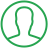# Write A Program To Print Fibonacci Series In Java

Last Updated : Jul 1, 2023In this article we will show you the solution of write a program to print Fibonacci series in java, Fibonacci numbers are formed by adding the previous number to its total. For instance, 0, 1, 1, 2, 3, 5, 8, 13, 21, 34, 55, etc

There are two ways to write a fibonacci series program in Java:

• Without recursion, use the Fibonacci Series
• Fibonacci Recursive Series

### Method 1

The method of iteration 1 is based on setting 0 and 1 as the initial and second numbers, respectively.

Once the initial number and second number are published, the third number will also be published.

### Method 2

Using Recursion: The sum of the two numbers before yields the Fibonacci numbers. Recursion can be used in the following situations:

• Obtain the numerical value necessary to calculate the Fibonacci series.
• Recursively iterate from N to 1:
• If a value is called repeatedly and the value is less than 1, the method defaults to returning 1.
• Recursive call: If the base case is in fact unsatisfied, call back for the preceding two values as
`recursive_function(N – 1) + recursive_function(N – 2);`

### Method 3

The repetitious work required by method 2 can be avoided by using dynamic programming to save the previously calculated Fibonacci numbers.

Steps are as follows :-

Create the arr N-dimensional array.

Set arr to 0 and arr to 1.

Modify the array arr[] by iterating through [2, N] as follows:

arr[i] = arr[i – 2] + arr[i – 1]

The sum of the first two digits in the Fibonacci sequence is the next digit. Nevertheless, if you are not familiar with Java concept of loops, stop reading right here.

## Step By Step Guide On Write A Program To Print Fibonacci Series In Java :-

```class TalkersCode{
public static void main(String args[])
{
int n1=0,n2=1,n3,i,count=10;
System.out.print(n1+" "+n2);
for(i=2;i<count;++i)
{
n3=n1+n2;
System.out.print(" "+n3);
n1=n2;
n2=n3;
}
}}```
1. First, we create the talkersCode class.
2. Then, the public static void main is defined.
3. Once 0 and 1 have been written, the loop moves on to 2, as well as the programme is terminated.

## Conclusion :-

We check for the two values that came before each fibonacci number for the sequence.

If we don't already know the answers to these values, we still use the same approach to discover them.

We will eventually discover that our needed index will continue to drop over time, and we will arrive at a point when we already know the answer.

We will continue creating all the index values we encountered before reaching the basic case using this value.

I hope this article on write a program to print fibonacci series in java helps you and the steps and method mentioned above are easy to follow and implement.

##About Riya

A recent graduate with a Bachelor of Technology (B.Tech) in Computer Science from India. She is passionate about leveraging technology to solve real-world problems. With a strong foundation and experience in programming languages such as Python, Django, HTML, CSS, and JavaScript, java, php and have honed her skills through hands-on projects and coursework.